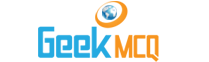Engineering :: Heat Transfer

1.  Transmission of heat by molecular collision is
 A. Conduction B. Convection C. Radiation D. Scattering E. Transmission

2.  Metals are good conductors of heat because
 A. They contain free electrons B. Their atoms are relatively far apart C. Their atoms collide frequently D. They have reflecting surfaces E. None of the above

3.  Heat is shown to be closely related to
 A. A fluid B. Energy C. Momentum D. Temperature E. Waveform

4.  Body A is kept in contact with body B. Heat will flow from A to B, if
 A. The heat content of A is greater than that of B B. The temperature of A is greter than that of B C. The specific heat of A is greater than that of B D. The specific heat of A is lower than that of B E. None of the above

5.  Cork is a good insulator because
 A. Its density is low B. It is porous C. It can be powdered D. It can be cast into rolls E. It is flexible

6.  Which one of the following cannot be unit of thermal conductivity?
 A. Watt / m2 K B. Kcal/m-hr?C C. BTU/ft-h?F D. cal/c,-sec?K E. CHU/ft-hr?C

7.  Which one of the following will have least value of thermal conductivity?
 A. Copper B. Silver C. Glass D. Water E. Air

8.  Which one of the following materials will have highest value of thermal conductivity?
 A. Steel B. Aluminium C. Brass D. Copper E. Lead

9.  Heat conduction does not occur
 A. If a physical body is impermeable to any kind of rays B. If the parts of a body are not in motion relative to one another C. If the bodies are kept in vacuum D. If the bodies are immersed in water E. If the temperature difference between the bodies does not exist

10.  Unsteady state of heat flow occurs in
 A. Flow of heat through furnace walls B. Flow of heat through insulated pipe with constant surface temperature C. Annealing of castings D. Flow of heat through refrigerator walls E. None of the above